Courses

# Maxwell's Equations in Linear Isotropic Media - Electromagnetic Theory, CSIR-NET Physical Sciences Physics Notes | EduRev

## Physics for IIT JAM, UGC - NET, CSIR NET

Created by: Akhilesh Thakur

## Physics : Maxwell's Equations in Linear Isotropic Media - Electromagnetic Theory, CSIR-NET Physical Sciences Physics Notes | EduRev

The document Maxwell's Equations in Linear Isotropic Media - Electromagnetic Theory, CSIR-NET Physical Sciences Physics Notes | EduRev is a part of the Physics Course Physics for IIT JAM, UGC - NET, CSIR NET.
All you need of Physics at this link: Physics

Maxwell’s equations are modified for polarized and magnetized materials.
For linear materials the polarization P and magnetization M is given by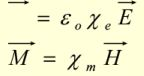And the D and B fields are related to E and H by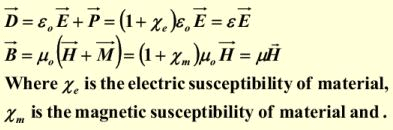Maxwell’s Equations Inside Matter (Cont’d)

• For polarized materials we have bound charges in addition to free charges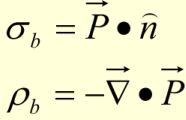• For magnetized materials we have bound currents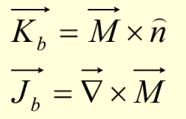• In electrodynamics any change in the electric polarization involves a flow of bound charges resulting in polarization current Js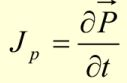Polarization current density is due to linear motion of charge when the Electric polarization changes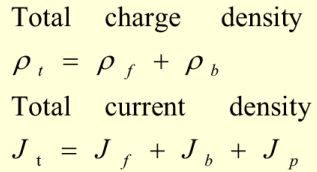•  Maxwell’s equations inside matter are written as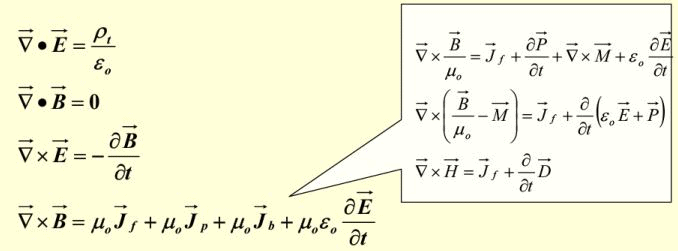• In non-dispersive, isotropic media  and µ are timeindependent scalars, and Maxwell’s equations reduces to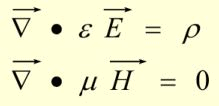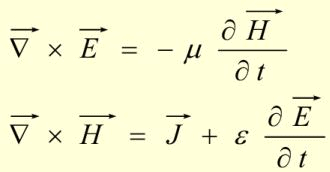• In uniform (homogeneous) medium ε and µ are independent of position, hence Maxwell’s equations reads as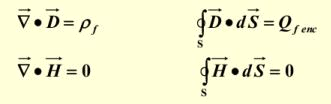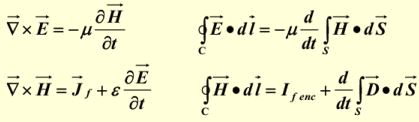Generally, ε and µ can be rank-2 tensor (3X3 matrices) describing birefringent anisotropic materials.

159 docs

,

,

,

,

,

,

,

,

,

,

,

,

,

,

,

,

,

,

,

,

,

,

,

,

;